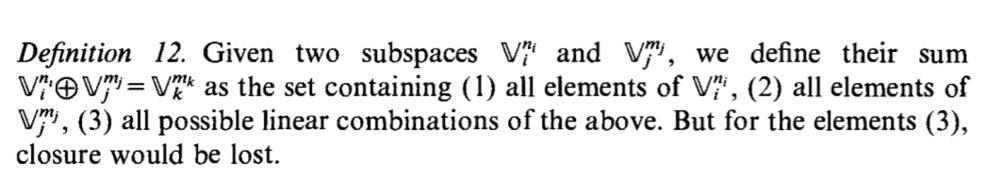# Closure in the subspace of linear combinations of vectors

• I
Summary:
Definition 12 in Principles of Quantum Mechanics, it says that when you have a subspace of vectors in 1 dimension, and another subspace of vectors in another dimension and finally a 3rd subspace with the linear combination of the first two; in the last case, closure will be lost. Why is that?This is the exact definition and I've summarized it, as I understand it above. Why is it, that for elements in the third subspace, closure will be lost? Wouldn't you still get another vector (when you add two vectors in that subspace), that's still a linear combination of the vectors in the first two subspaces? So, if the first subspace is of all vectors in the x_hat direction and the second subspace is of vectors in the y_hat direction. Then the third subspace pretty contains all the vectors in 2D and when you add two of them, it will give you another, that can always be written as a linear combination of x_hat and y_hat. What am I missing here?

## Answers and Replies

George Jones
Staff Emeritus
Science Advisor
Gold Member
Summary:: Definition 12 in Principles of Quantum Mechanics, it says that when you have a subspace of vectors in 1 dimension, and another subspace of vectors in another dimension and finally a 3rd subspace with the linear combination of the first two; in the last case, closure will be lost. Why is that?

View attachment 266632

This is the exact definition and I've summarized it, as I understand it above. Why is it, that for elements in the third subspace, closure will be lost? Wouldn't you still get another vector (when you add two vectors in that subspace), that's still a linear combination of the vectors in the first two subspaces? So, if the first subspace is of all vectors in the x_hat direction and the second subspace is of vectors in the y_hat direction. Then the third subspace pretty contains all the vectors in 2D and when you add two of them, it will give you another, that can always be written as a linear combination of x_hat and y_hat. What am I missing here?

I think this is a question about English, not math. "But for the elements (3)" should be read as "If not for the elements (3)".

•fresh_42
I think this is a question about English, not math. "But for the elements (3)" should be read as "If not for the elements (3)".
Ah, I see, thanks! LOL